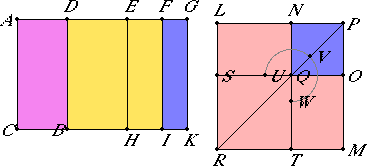# Proposition 94

If an area is contained by a rational straight line and a fourth apotome, then the side of the area is minor.

Let the area be contained by the rational straight line AC and the fourth apotome AD.

I say that the side of the area AB is minor.X.Def.III.4

Let DG be the annex to AD, therefore AG and GD are rational straight lines commensurable in square only, AG is commensurable in length with the rational straight line AC set out, and the square on the whole AG is greater than the square on the annex DG by the square on a straight line incommensurable in length with AG.

X.18

Since the square on AG is greater than the square on GD by the square on a straight line incommensurable in length with AG, therefore, if there is applied to AG a parallelogram equal to the fourth part of the square on DG and deficient by a square figure, then it divides it into incommensurable parts.

Bisect DG at E, apply to AG a parallelogram equal to the square on EG and deficient by a square figure, and let it be the rectangle AF by FG. Then AF is incommensurable in length with FG.

Draw EH, FI, and GK through E, F, and G parallel to AC and BD.

X.19

Since AG is rational and commensurable in length with AC, therefore the whole AK is rational.

X.21

Again, since DG is incommensurable in length with AC, and both are rational, therefore DK is medial.

Again, since AF is incommensurable in length with FG, therefore AI is incommensurable with FK.

Now construct the square LM equal to AI, and subtract NO, equal to FK, about the same angle, the angle LPM.

VI.26

Therefore the squares LM and NO are about the same diameter. Let PR be their diameter, and draw the figure.

VI.17

Since the rectangle AF by FG equals the square on EG, therefore, AF is to EG as EG is to FG.

But AF is to EG as AI is to EK, and EG is to FG as EK is to FK, therefore EK is a mean proportional between AI and FK.

But MN is also a mean proportional between the squares LM and NO, and AI equals LM, and FK equals NO therefore EK also equals MN.

But DH equals EK, and LO equals MN, therefore the whole DK equals the gnomon UVW and NO.

Since, then, the whole AK equals the sum of the squares LM and NO, and, in these, DK equals the gnomon UVW and the square NO, therefore the remainder AB equals ST, that is, to the square on LN. Therefore LN is the side of the area AB.

I say that LN is the irrational straight line called minor.

Since AK is rational and equals the sum of the squares on LP and PN, therefore the sum of the squares on LP and PN is rational.

Again, since DK is medial, and DK equals twice the rectangle LP by PN, therefore twice the rectangle LP by PN is medial.

And, since AI was proved incommensurable with FK, therefore the square on LP is also incommensurable with the square on PN.

Therefore LP and PN are straight lines incommensurable in square which make the sum of the squares on them rational, but twice the rectangle contained by them medial.

X.76

Therefore LN is the irrational straight line called minor, and it is the side of the area AB.

Therefore the side of the area AB is minor.

Therefore, if an area is contained by a rational straight line and a fourth apotome, then the side of the area is minor.

Q.E.D.

## Guide

This proposition is used in X.108. It is also used in proposition XIII.11.